Common Fixed Point Theorem in T0 Quasi Metric Space | OMICS International
Fluid Mechanics: Open Access

# Common Fixed Point Theorem in T0 Quasi Metric Space

Balaji R Wadkar1, Ramakant Bhardwaj2, Lakshmi Narayan Mishra3* and Basant Singh1

2Department of Mathematics, TIT Group of Institutes, Anand Nagar, Bhopal, Madhya Pradesh, India

3Department of Mathematics, Mody University of Science and Technology, Lakshmangarh, Sikar Road, Sikar, Rajasthan, India

*Corresponding Author:
Mishra LN
Department of Mathematics
Mody University of Science and Technology
Rajasthan 332 311, India
Tel: +919913387604
E-mail: [email protected]

Received Date: January 02, 2017; Accepted Date: January 30, 2017; Published Date: February 08, 2017

Citation: Wadkar BR, Bhardwaj R, Mishra LN, Singh B (2017) Common Fixed Point Theorem in T0 Quasi Metric Space. Fluid Mech Open Acc 4: 143. doi: 10.4172/2476-2296.1000143

Copyright: © 2017 Wadkar BR, et al. This is an open-access article distributed under the terms of the Creative Commons Attribution License, which permits unrestricted use, distribution, and reproduction in any medium, provided the original author and source are credited.

Visit for more related articles at Fluid Mechanics: Open Access

#### Abstract

In this paper, we prove fixed point theorems for generalized C-contractive and generalized S-contractive mappings in a bi-complete di-metric space. The relationship between q- spherically complete T0 Ultra-quasi-metric space and bi-complete diametric space is pointed out in proposition 5.1. This work is motivated by Petals and Fvidalis in a T0-ultraquasi- metric space 2000 AMS Subject Classification: 47H17, 74H05, 47H09.

#### Keywords

Fixed point; Generalized C-contraction; Generalized S-contraction; Spherically complete; Bi-complete di-metric

#### Introduction

In Agyingi  proved that every generalized contractive mapping defined in a q- spherically complete T0-ultra-quasi metric space has a unique fixed point. In Petals and Fvidalis  proved that every contractive mapping on a spherically complete non Archimedean normed space has a unique fixed point. Agyingi and Gega proved fixed point theorems in a T0-ultra-quasi-metric space [3-6]. Later many authors published number of papers in this space [7-10].

In this paper we shall prove a fixed point theorem for generalized c- contractive and generalized s-contractive mappings in a bi-complete di-metric space.

If we delete, in the used definition of the pseudo metric d on the set X. the symmetry condition, d(x, y)=d(y, x), whenever x, y ∈ X we are led to the concept of quasi-pseudo metric.

Definition 1.1: Let (X ,m) be a metric space. Let T: X→ X map is called a C-contraction if there exist,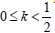such that for all x, y ∈ X the following inequality holds ,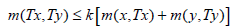Definition 1.2: Let (X,m) be a metric space. A map T: X→ X is called a S-contraction if there exist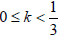such that for all x, y ∈ X the following inequality holds ,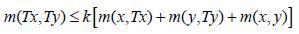Preliminaries

Now we recall some elementary definitions and terminology from the asymmetric topology which are necessary for a good understanding of the work below.

Definition 4.1: Let X be a non empty set. A function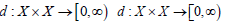is called quasi pseudo metric on X if

d(x, x) = 0,∀x∈ X

d(x, z) ≤ d(x, y) + d( y, z),∀x, y, z∈ X

Moreover if d(x, y) = 0 = d( y, x)⇒ x = y then d is said to be a T0 quasi metric or di-metric. The latter condition is referred as the T0 condition.

Example 4.1: On R×R, we define the real valued map d given by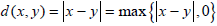then (R,d) is a di metric space.

Remark 4.1

Let d be quasi-pseudo metric on X, then the map d-1 defined by d-1 (x, y)=d(y, x) whenever x, y X is also a quasi-pseudo metric on X, called the conjugate of d.

It is also denoted by dt or d. It is easy to verify that the function ds defined by ds=d∨d-1

i.e.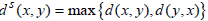defines a metric on X whenever d is a T0 quasi pseudo metric.

In some cases, we need to replace [0, ∞) by [0, ∞) (where for a d attaining the value ∞, the triangle inequality is interpreted in the obvious way). In such case we speak of extended quasi- pseudo metric.

Definition 4.3: The di metric space (X, d) is said to be bi complete if the metric space (R, ds) is complete.

Example 4.2: Let X=[0, ∞) define for each x, y∈ X , n(x, y) = x if x > y and n(x, y) = 0 if x < y . It is not difficult to check that (X,n) is a T0 quasi pseudo metric space . Notice that, for x, y∈[0,∞) , we have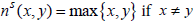and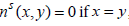the matrix ns is complete on (X ,d).

Definition 4.3: Let (X ,d) be quasi pseudo metric space, for x,∈ X&∈> 0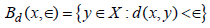denotes the open ∈ − ball at x. The collection of such balls is a base for a topology τ(d) induced by d on X. Similarly for x,∈ X&∈≥ 0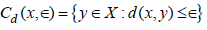denotes the closed ∈ − ball at x.

Definition 4.4: Let (X ,d) be quasi pseudo metric space Let (xi),i∈I be a family of points in X and let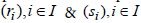be a family of non negative real numbers.

We say that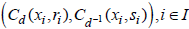has the mixed binary intersection property provided that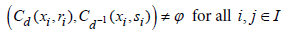Definition 4.5: Let (X ,d) be quasi pseudo metric space we say that (X ,d) is Isbell complete provided that each family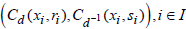that has the mixed binary intersection property is such that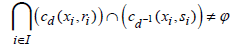Proposition 4.1: If (X ,d) is an extended Isbell-complete quasipseudo metric space then (X ,ds) is hyper complete. An interesting class of quasi pseudo metric space, for which, we investing a type of completeness are the ultra quasi pseudo metric.

Definition 4.6: Let X be a set & d : X × X →[0,∞) be function a function mapping into the set [0,∞) of non negative real’s then d is ultra quasi pseudo metric on X if

d(x, x) = 0 for all x in X &

d(x, z) ≤ max{d(x, y),d( y, z)} whenever x, y, z∈ X

The conjugate d–1 of d where d–1(x, y) = d( y, x) whenever x, y∈ X is also an ultra quasi pseudo metric on X.

If d also satisfies the T0 – condition, then d is called a T0- ultra quasi metric on X. Notice that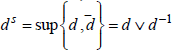is an ultra-metric on X whenever d is a T0- ultra quasi metric.

In a literature, T0- ultra quasi metric spaces are also known as non Archimedean T0- quasi metric.

q-spherically Completeness

In this section we shall recall some results about q- spherical completeness belonging mainly to .

Definition 5.1: Let (X ,d) be an ultra–quasi pseudo metric space Let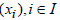be a family of points in X and let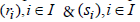be (X ,d) is q- spherical complete provided that each family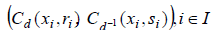Satisfying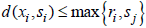, whenever i, j∈I is such that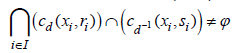Proposition 5.1: Each q- spherically complete T0 ultra quasi metric space (X, d) is bi-complete .

#### Main Results

We recall the following interesting results respectively due to Chatterji  and to Shukla 

Theorem 6.a A C- contraction on a complete metric space has a unique fixed point .

Theorem 6.b A S- contraction on a complete metric space has a unique fixed point 

Following results generalizes the above theorem to setting of a bicomplete di-metric space.

Definition 6.1: Let (X ,d) be a quasi pseudo metric space. A map T : X → X is called a c-pseudo contraction if there exist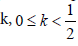such that for all x, y ∈ X the following inequality holds.

d(Tx,Ty) ≤ k [d(Tx, x) + d( y,Ty)]

Definition 6.2: Let (X ,d) be a quasi-pseudo metric space. A map T : X → X is called a S-pseudo contraction if there exist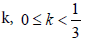such that for all x, y ∈ X the following inequality holds.

d(Tx,Ty) ≤ k [d(Tx, x) + d( y,Ty) + d(x, y)]

Now we define following definitions

Definition 6.3: Let (X ,d) be a quasi-pseudo metric space. A map T : X → X is called a generalized c-pseudo contraction if there exist k,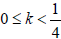such that for all x, y ∈ X the following inequality holds.Definition 6.4: Let (X ,d) be a quasi-pseudo metric space. A map T : X → X is called a generalized S-pseudo contraction if there exist k,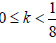such that for all x, y ∈ X the following inequality holds.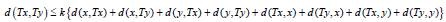Theorem 6.1:

Let (X ,d) be a bi complete di metric space and let T : X → X be a generalized c- pseudo contraction then T has a unique fixed point.

Proof: Since T : X → X is a generalized c-pseudo contraction then there exist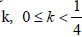such that for all x, y∈ X the following inequality holds:

d(Tx,T) ≤ k{d(,Tx, x) + d( y,Ty) + d(Tx, y) + d(x,Ty)}

We shall first show that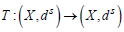is a generalized ccontraction.

Since for any x, y ∈ X we have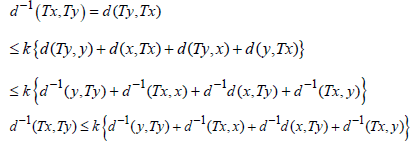We see that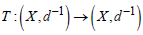is a generalized C-pseudo contraction therefore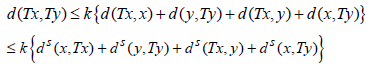and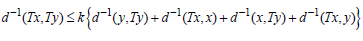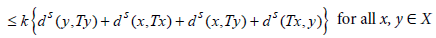Hence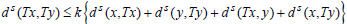, for all x, y ∈ X

and so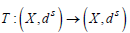is a generalized C- contraction.

By assumption (X,d) is a bi complete. Hence (X,ds) is complete. There fore by theorem (4a) T has a unique fixed point. This completes the proof.

Corollary 6.1: Let (X,d) be a T0-Isbell-Complete quasi pseudo metric spaces and T : X → X be a generalized c-pseudo contraction then T has a unique fixed point.

The proof follows from the proposition 2.1

Corollary 6.2: Any generalized c- pseudo contraction on a q-spherically complete T0 ultra quasi metric space has a unique fixed point.

The proof follows from the proposition 3.1

Theorem 6.2:

Let (X,d) be a bi complete di metric space and let T : X → X be an generalized S pseudo contraction then T has a unique fixed point

Proof: As in the previous proof it is enough to prove that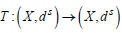is an generalized S –contraction.

Since T : X → X be a S –pseudo contraction then there exist k,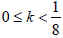such that for all x, y ∈ X the following inequality holds: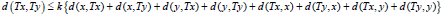We shall first show that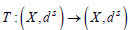is a generalized Ccontraction.

Since for any x, y ∈ X we have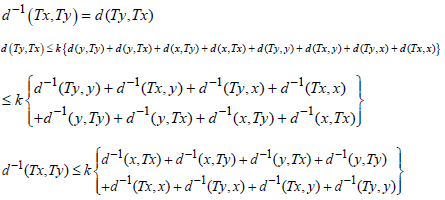We see that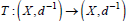is a pseudo contraction.

Therefore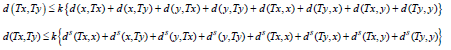and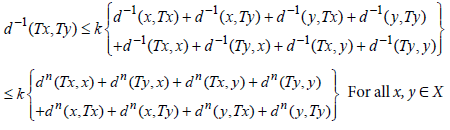Hence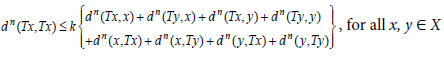and so T : (X,ds)→(X,ds) is a generalized s-contraction.

By assumption (X,d) is a bi complete. Hence (X,ds) is complete. There fore by theorem (4a) T has a unique fixed point. This completes the proof.

Corollary 6.3: Let ( X ,d ) be a T0-Isbell-Complete quasi pseudo metric spaces and T : X → X be a pseudo contraction then T has a unique fixed point.

The proof follows from the proposition 2.1

Corollary 6.4: Any s-pseudo contraction on a q-spherically complete T0 ultra quasi metric space has a unique fixed point.

#### References

Select your language of interest to view the total content in your interested language

### Article Usage

• Total views: 1753
• [From(publication date):
February-2017 - Dec 13, 2019]
• Breakdown by view type
• HTML page views : 1580Can't read the image? click here to refresh